# ReadMe!!!!

Viewing posts from October, 2007

## Miguel de Unamuno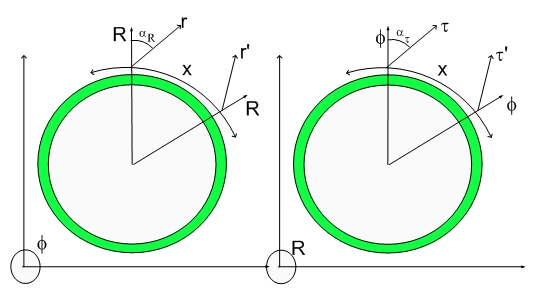"Only he who attempts the absurd is capable of achieving the impossible"
Miguel de Unamuno

I couldn't resist....:) Check the
interview. It is quite funny....

Well, well... We are experiencing Technical Difficulties and you might have to go to the link itself until I figure out how to put a video in this blog...:)

In fact, I heard that Presidential Candidate Dennis Kucinich also carries a pocket copy of my Vacuum Permittivity Formula...:)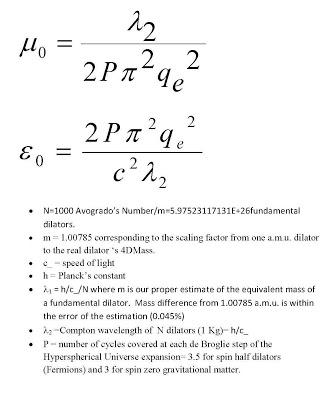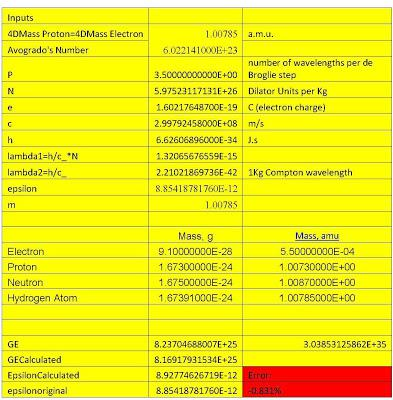I forgot to mention, another source of error might be Wikipedia...:) All my numbers comes from that handy source...:)

There is also a numerically invisible 1Kg on the denominator of mu and in the numerator of epsilon, otherwise the units would be incorrect. Please check the derivation on the pdf for details.

The error in the estimation of the Vacuum Permittivity is 0.008:1 or
0.8%

I hope you people are smart enough to understand that the error estimate in this case also means that conversely there is a 0.8% possible error in the estimation of the fundamental dilator 4DMass or more precisely there is a total propagated error of 0.8% from all the sources...:) Remember that I use the preferential reference frame to derive the forces. I didn't take Gravitation or absolute speed in consideration.

Even the most retarded editor/moderator etc would be impressed with those numbers...:) after all this is a quantity that is IMPOSSIBLE to be calculated...:0

I am still awaiting for Stephen Hawking's reply...:)

Cheers,

MP

## Hypergeometrical EpsilonHow to calculate Cosmological Constants

The vacuum permitivity is a Cosmological Constant, that is, there is NO formula for it. That might be the case in other theories.

In fact, this is a great time for a DARE.... :) Just to check if you people are awaken or not...:) Go ahead and ask Stephen Hawking if he knows how to calculate THE PERMITTIVITY OF VACUUM....:)

I bet money he doesn't...:)

Well, I am trying to emphasize how different my theory is from M Theory, String Theory or any other.... In any other theory, someone would present some Lagrangian, Metric for some wildly guessed scenario (e.g. branes under Gravitation interaction)...:) and from that imaginary Universe they would extract some impossible to measure results.... Not too long ago someone calculated that the Gravitational fields between branes decays exponentially... while decaying inversely with the square of distance within the brane...:) At the time, this was heralded as a challenge to Einstein and/or Newton... I thought to myself, Ginsparg has the petulance to preclude me from using a megabyte of hard drive on his Cornell-Los Alamos Arxives... and this piece of speculative science is hailed as great science...

I should say that I am only mentioning this specific case because I can remember it...

## So much for WikipediaWikipedia Entry Set for Speedy Deletion -

I considered writing a small posting on Wikipedia to explain the Fundamental Dilator Paradigm. This is a fundamental part of my theory and the link (hidden variable) that allow one goes from Classical Mechanics to Quantum Mechanics...:)

I wrote the following little thing:

== Fundamental Dilator in the Hypergeometrical Universe Model ==

In the Hypergeometrical Universe Model,“particles” are considered to be coherences between 4D stationary states of metric deformation. These coherence can have spin (rotation along any axis perpendicular to R), and nuclear angular momentum (rotation around any axis in the Fabric of Space).

The Fabric of Space (FS) is defined within the Hypergeometrical Universe Model as the lightspeed traveling locus where all the dilators are located. This corresponds to a 3D hypersurface on non-compact Cartesian four-dimensional space. This lightspeed expanding hypersurface contains our 3D Universe.

Local deformations of the Fabric of Space are achieved by dilator dilaton field interactions which result in acceleration and eventually in constant velocity lateral travel. The inertial motion is how the Fabric of Space relaxes. Dilators will travel until they reach their Hubble angle. This is the Hypergeometrical Universe Newton’s First Law.

Since the boundary where the Universe (3D) is located is moving at the speed of light along R, the Hypergeometrical Universe Model does not contain the concept of particle since that doesn’t include the inherent lighspeed motion and has inherently some immutability assumptions.

The dilator is by definition mobile and always tunneling back and forth. It can have non-zero spin. In the Hypergeometrical Standard Model presented in the Hypergeometrical Universe blog, all particles models are shown to be decomposable into variations of the fundamental dilator. Different phases of the fundamental dilator correspond to Proton, Electron, Positron and antiproton.

There is an obvious analogy to musical notes. Each dilator phase (proton, electron, antiproton and positron) can be thought as dimensional notes (notes that modulate the space metric as opposed to air density).

Electron Model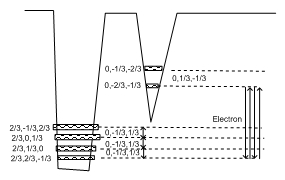Figure 1. 4D Stationary Deformation State diagram for electron

The coherence four notes repeat forever.
Where p=(2/3,2/3,-1/3), p*=(2/3,-1/3,2/3),e=(0,-2/3,-1/3),e*=(0,-1/3,-2/3) are a subset of states involved in the most common “particles”= proton, electron and neutron, isotopes, hyperons.

Below is another representation of the electron and positron. Notice that the first and last elements of the coherence chain are the same and that the coherence repeats itself for its lifetime. In the case of a proton/electron, that lifetime is infinite, since that coherence is between two ground states.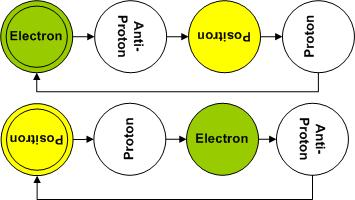Figure 2. Dimensional notes associated with Electron and Positron.
Yellow (ligther)= positive charge.
Green (darker)= Negative charge.
White = Invisible in 3D due to 4D orientation – perpendicular to the Fabric of Space.

This is an effort to represent a tumbling 4D object, which changes shape as it tumbles. Notice that the sidewise states have no FS overlap. Interaction or “Existence” depends upon FS overlap and the frequency matching with the other dilators. This is the reason behind representing all “particle” in terms of the Fundamental Dilator or the Hypergeometrical Standard Model.

Since in the theory, there is an absolute time, one can define an absolute phase and that is what distinguishes an electron from a positron. Later it will be clear that more complex coherences involving the p* and e* state (neutrino states) will result in a phase shift of the tunneling process with respect to the tumbling process, thus modifying which state is in phase with the shock-wave universe.

The colors are shown only for states that have both a FS overlap and the same frequency as the fundamental dilator.

Another important element of the model is the bolding of the third axis length (e.g. p=(2/3,2/3,-1/3)). This means that the spin is a tumbling process around and rotational axis perpendicular to both the radial direction (perpendicular to all three spatial coordinates and the z coordinate). This defines a 4D angular momentum, which has to be conserved. More complex coherences like the ones associated with Delta and Sigma particles differs just by the final spin and thus by how the sub-coherences tumbles to make up the final amount of spinning.

Proton Model

Similarly for a proton: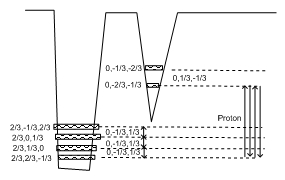Figure 3. 4D Stationary Deformation State diagram for proton

The coherence’s four notes repeat forever, thus the fourth state is represented connecting to the initial state emphasizing the closing of the coherence cycle. Belly up states represent anti-states (anti-proton or positron states).

Here is the representation of a proton and an antiproton.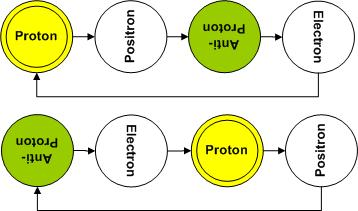Figure 4. Dimensional notes associated with Proton and anti-Proton.

The fundamental dilator is the paradigm behind the Fat Electron (4DMass=1.004145287 a.m.u. electron) used in the derivations of Electrostatic and Biot-Savart Laws in the Hypergeometrical Universe Model.

3D and 4D Masses

The Hypergeometrical Universe Model is a purely geometrical theory. This means that there is no concept of Mass, Charge, Force. Instead of Mass one has 4D displacement volumes (4DMasses) and their equivalent 3D FS Overlap displacement volumes (3DMasses).

This means that the dilaton field (lighspeed traveling dilatons) are coherently generated by all the four phases of the fundamental dilator and thus sense the equivalent volume of a proton and an electron. This means that the 4D Mass of Proton,Antiproton,Electron and Positron are the same.

Newton’s :Law of Gravitation, Gauss Law of Electrostatics and Biot-Savart Law of Magnetism were rederived from first principles as a law guiding dilator motion subject to a four-dimensional dilaton field. A Quantum Lagrangian Principle was proposed based on the minimization of virtual work done by constraints (FS). This QLP states that a dilator will step through the de Broglie expansion always in phase with the surrounding dilaton field.

Hence, 3DMass is the overlap of the fundamental dilator displacement volume with the FS.

The 4DMass can be understood as a mass proportional to the 4D volume associated with a dilator cycle. In a dilator cycle one has the two phases of an electron and the two phases of a proton, thus resulting in a coherent displacement 4D volume of a proton and an electron. The ideal 4DMass would be 1.00785 a.m.u.. The discrepancy is the result that the 4D space is not totally isotropic, that is, the shock-wave universe creates a small anisotropy that makes radial and FS orientations different in terms of displacement volumes of coherent states.

Below is the corresponding Balls Diagram for the Neutron and the Hydrogen Atom.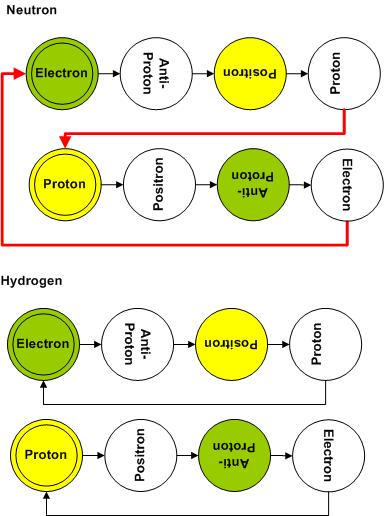Figure 5. This figure displays the Neutron and Hydrogen Atom diagrams. Notice the red lines corresponding to the transmutation notes. These transmutation notes correspond to 3D rotations of the 4D displacement volume at specific phases of the dilator coherence.

The Hypergeometrial Model is based on the following diagram:Figure 6. This figure shows the two cross-sections representing the Hypergeometrical Universe Model. The 3D Universe topology is one of a four-dimensional shockwave traveling at the speed of light perpendicular to our space.

==References==

Quantization in Astrophysics, Brownian Motion, and Supersymmetry (2007) editors: Florentin Smarandache; V. Christianto ISBN 819021909X
--------------------------------------------------------------------------
--------------------------------------------------------------------------

## How to Write a Theory of EverythingHow to Write a Theory of Everything

It turns out that writing a theory of everything is not a simple as you might think. The first reason being that every scientist would hate to have someone else writing it before them..:) That explains some of the reactionary responses to my efforts to disseminate my ideas.

The second reason is the necessarily wide scope of the writing. A theory of everything has to replicate all data associated with Cosmology, our concepts of Cosmogenesis, Quantum Mechanics and Particle Physics (preferably in compliance with the Standard Model and written in terms of Strings or Branes)..:) After all it is just common cortesy to write it in the language of the people who will read it...:)

## Hypergeometrical Universe EpsilonHow to calculate Cosmological Constants

RSS / Atom